Search by Topic

Resources tagged with Visualising similar to Quad Match:

Filter by: Content type:
Age range:
Challenge level:

There are 261 resultsInside Seven Squares

Age 7 to 11 Challenge Level:

What is the total area of the four outside triangles which are outlined in red in this arrangement of squares inside each other?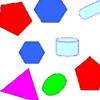Part the Polygons

Age 7 to 11 Short Challenge Level:

Draw three straight lines to separate these shapes into four groups - each group must contain one of each shape.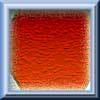Hexagon Transformations

Age 7 to 11 Challenge Level:

Can you cut a regular hexagon into two pieces to make a parallelogram? Try cutting it into three pieces to make a rhombus!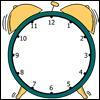A Square in a Circle

Age 7 to 11 Challenge Level:

What shape has Harry drawn on this clock face? Can you find its area? What is the largest number of square tiles that could cover this area?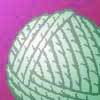Age 7 to 11 Challenge Level:

This practical problem challenges you to make quadrilaterals with a loop of string. You'll need some friends to help!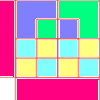Diagrams

Age 7 to 11 Challenge Level:

A group activity using visualisation of squares and triangles.Baravelle

Age 7 to 16 Challenge Level:

What can you see? What do you notice? What questions can you ask?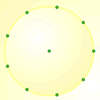Age 7 to 11 Challenge Level:

How many DIFFERENT quadrilaterals can be made by joining the dots on the 8-point circle?Getting an Angle

Age 11 to 14 Challenge Level:

How can you make an angle of 60 degrees by folding a sheet of paper twice?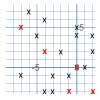Ten Hidden Squares

Age 7 to 11 Challenge Level:

These points all mark the vertices (corners) of ten hidden squares. Can you find the 10 hidden squares?Counting Triangles

Age 11 to 14 Challenge Level:

Triangles are formed by joining the vertices of a skeletal cube. How many different types of triangle are there? How many triangles altogether?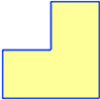Twice as Big?

Age 7 to 11 Challenge Level:

Investigate how the four L-shapes fit together to make an enlarged L-shape. You could explore this idea with other shapes too.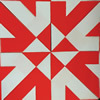Folding Flowers 1

Age 7 to 11 Challenge Level:

Can you visualise what shape this piece of paper will make when it is folded?Sea Defences

Age 7 to 14 Challenge Level:

These are pictures of the sea defences at New Brighton. Can you work out what a basic shape might be in both images of the sea wall and work out a way they might fit together?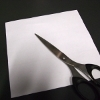Square to L

Age 7 to 11 Challenge Level:

Find a way to cut a 4 by 4 square into only two pieces, then rejoin the two pieces to make an L shape 6 units high.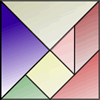Age 7 to 11 Challenge Level:

How can the same pieces of the tangram make this bowl before and after it was chipped? Use the interactivity to try and work out what is going on!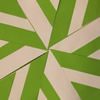Folding Flowers 2

Age 7 to 11 Challenge Level:

Make a flower design using the same shape made out of different sizes of paper.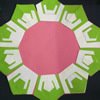Regular Rings 2

Age 7 to 11 Challenge Level:

What shape is made when you fold using this crease pattern? Can you make a ring design?LOGO Challenge - Circles as Animals

Age 11 to 16 Challenge Level:

See if you can anticipate successive 'generations' of the two animals shown here.Putting Two and Two Together

Age 7 to 11 Challenge Level:

In how many ways can you fit two of these yellow triangles together? Can you predict the number of ways two blue triangles can be fitted together?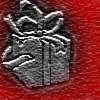Wrapping Presents

Age 7 to 11 Challenge Level:

Choose a box and work out the smallest rectangle of paper needed to wrap it so that it is completely covered.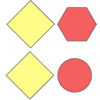Overlapping Again

Age 7 to 11 Challenge Level:

What shape is the overlap when you slide one of these shapes half way across another? Can you picture it in your head? Use the interactivity to check your visualisation.Nine-pin Triangles

Age 7 to 11 Challenge Level:

How many different triangles can you make on a circular pegboard that has nine pegs?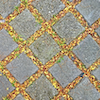Seeing Squares

Age 5 to 11 Challenge Level:

Players take it in turns to choose a dot on the grid. The winner is the first to have four dots that can be joined to form a square.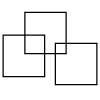Three Squares

Age 5 to 11 Challenge Level:

What is the greatest number of squares you can make by overlapping three squares?The Old Goats

Age 11 to 14 Challenge Level:

A rectangular field has two posts with a ring on top of each post. There are two quarrelsome goats and plenty of ropes which you can tie to their collars. How can you secure them so they can't. . . .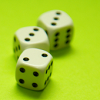Dicey

Age 7 to 11 Challenge Level:

A game has a special dice with a colour spot on each face. These three pictures show different views of the same dice. What colour is opposite blue?Efficient Cutting

Age 11 to 14 Challenge Level:

Use a single sheet of A4 paper and make a cylinder having the greatest possible volume. The cylinder must be closed off by a circle at each end.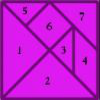The Development of Spatial and Geometric Thinking: the Importance of Instruction.

Age 5 to 11

This article looks at levels of geometric thinking and the types of activities required to develop this thinking.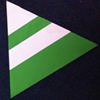Regular Rings 1

Age 7 to 11 Challenge Level:

Can you work out what shape is made by folding in this way? Why not create some patterns using this shape but in different sizes?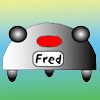Fred the Class Robot

Age 7 to 11 Challenge Level:

Billy's class had a robot called Fred who could draw with chalk held underneath him. What shapes did the pupils make Fred draw?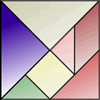World of Tan 6 - Junk

Age 7 to 11 Challenge Level:

Can you fit the tangram pieces into the silhouette of the junk?Constructing Triangles

Age 11 to 14 Challenge Level:

Generate three random numbers to determine the side lengths of a triangle. What triangles can you draw?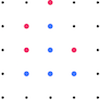Square Surprise

Age 5 to 11 Challenge Level:

Why do you think that the red player chose that particular dot in this game of Seeing Squares?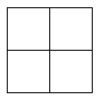Two Squared

Age 7 to 11 Challenge Level:

What happens to the area of a square if you double the length of the sides? Try the same thing with rectangles, diamonds and other shapes. How do the four smaller ones fit into the larger one?Triangles in the Middle

Age 11 to 18 Challenge Level:

This task depends on groups working collaboratively, discussing and reasoning to agree a final product.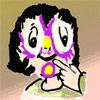Painted Faces

Age 7 to 11 Challenge Level:

Imagine a 3 by 3 by 3 cube made of 9 small cubes. Each face of the large cube is painted a different colour. How many small cubes will have two painted faces? Where are they?Framed

Age 11 to 14 Challenge Level:

Seven small rectangular pictures have one inch wide frames. The frames are removed and the pictures are fitted together like a jigsaw to make a rectangle of length 12 inches. Find the dimensions of. . . .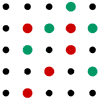Seeing Squares for Two

Age 5 to 11 Challenge Level:

Seeing Squares game for an adult and child. Can you come up with a way of always winning this game?Trice

Age 11 to 14 Challenge Level:

ABCDEFGH is a 3 by 3 by 3 cube. Point P is 1/3 along AB (that is AP : PB = 1 : 2), point Q is 1/3 along GH and point R is 1/3 along ED. What is the area of the triangle PQR?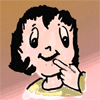Cube Drilling

Age 7 to 11 Challenge Level:

Imagine a 4 by 4 by 4 cube. If you and a friend drill holes in some of the small cubes in the ways described, how many will not have holes drilled through them?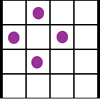Square Corners

Age 7 to 11 Challenge Level:

What is the greatest number of counters you can place on the grid below without four of them lying at the corners of a square?Age 11 to 14 Challenge Level:

Four rods, two of length a and two of length b, are linked to form a kite. The linkage is moveable so that the angles change. What is the maximum area of the kite?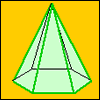A Chain of Eight Polyhedra

Age 7 to 11 Challenge Level:

Can you arrange the shapes in a chain so that each one shares a face (or faces) that are the same shape as the one that follows it?Square It

Age 11 to 16 Challenge Level:

Players take it in turns to choose a dot on the grid. The winner is the first to have four dots that can be joined to form a square.Nine Colours

Age 11 to 16 Challenge Level:

Can you use small coloured cubes to make a 3 by 3 by 3 cube so that each face of the bigger cube contains one of each colour?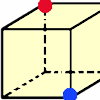Redblue

Age 7 to 11 Challenge Level:

Investigate the number of paths you can take from one vertex to another in these 3D shapes. Is it possible to take an odd number and an even number of paths to the same vertex?Age 11 to 14 Challenge Level:

Choose a couple of the sequences. Try to picture how to make the next, and the next, and the next... Can you describe your reasoning?Multiplication Series: Illustrating Number Properties with Arrays

Age 5 to 11

This article for teachers describes how modelling number properties involving multiplication using an array of objects not only allows children to represent their thinking with concrete materials,. . . .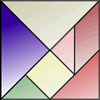World of Tan 14 - Celebrations

Age 7 to 11 Challenge Level:

Can you fit the tangram pieces into the outline of Little Ming and Little Fung dancing?